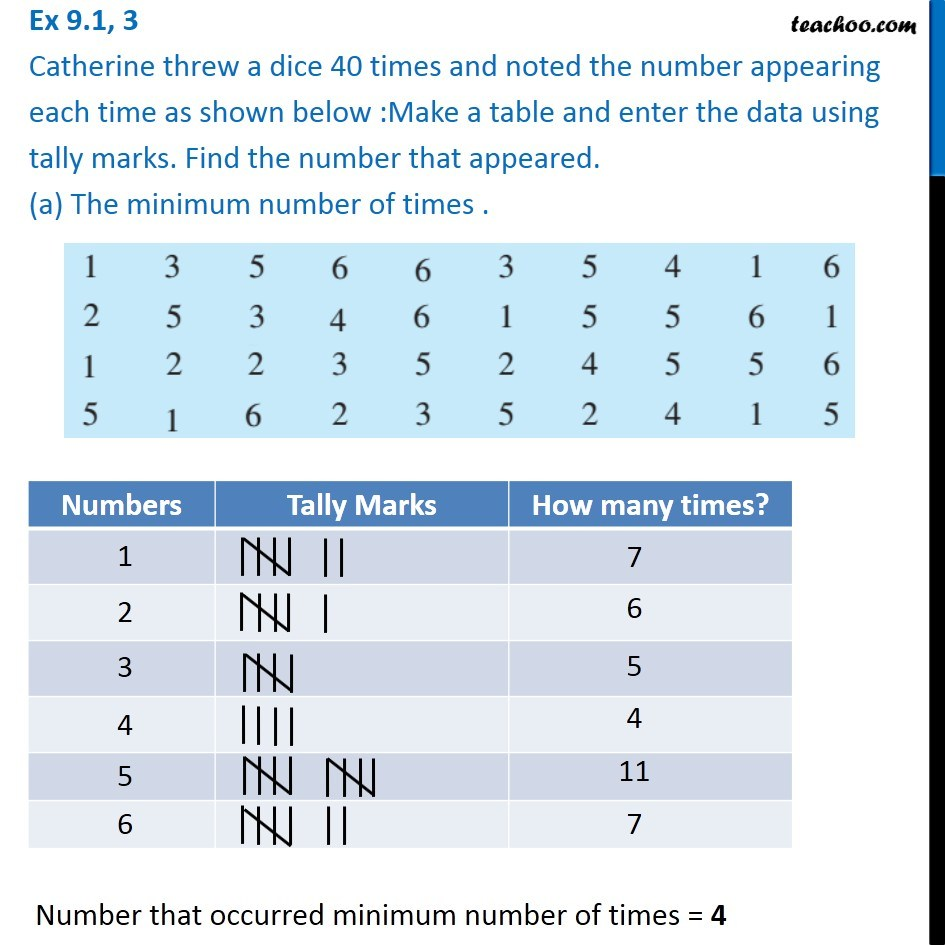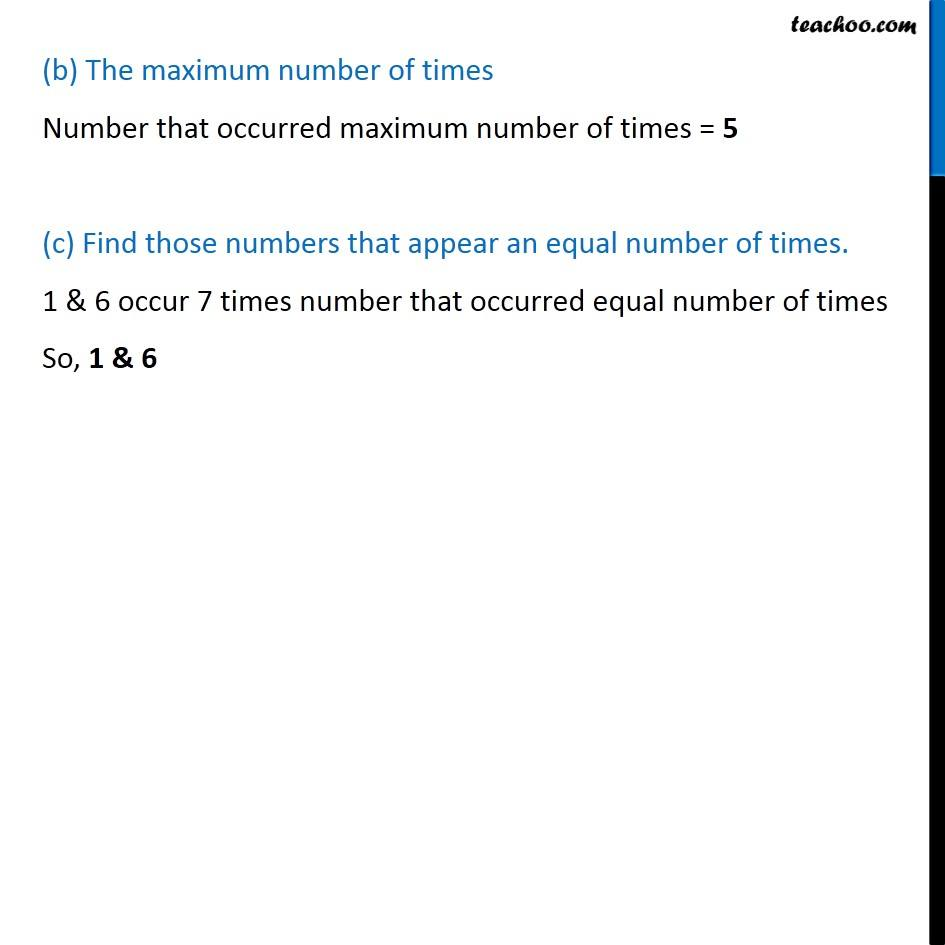Ex 9.1

Chapter 9 Class 6 Data Handling
Serial order wiseLearn in your speed, with individual attention - Teachoo Maths 1-on-1 Class

### Transcript

Ex 9.1, 3 Catherine threw a dice 40 times and noted the number appearing each time as shown below :Make a table and enter the data using tally marks. Find the number that appeared. (a) The minimum number of times . Number that occurred minimum number of times = 4 (b) The maximum number of times Number that occurred maximum number of times = 5 (c) Find those numbers that appear an equal number of times. 1 & 6 occur 7 times number that occurred equal number of times So, 1 & 6